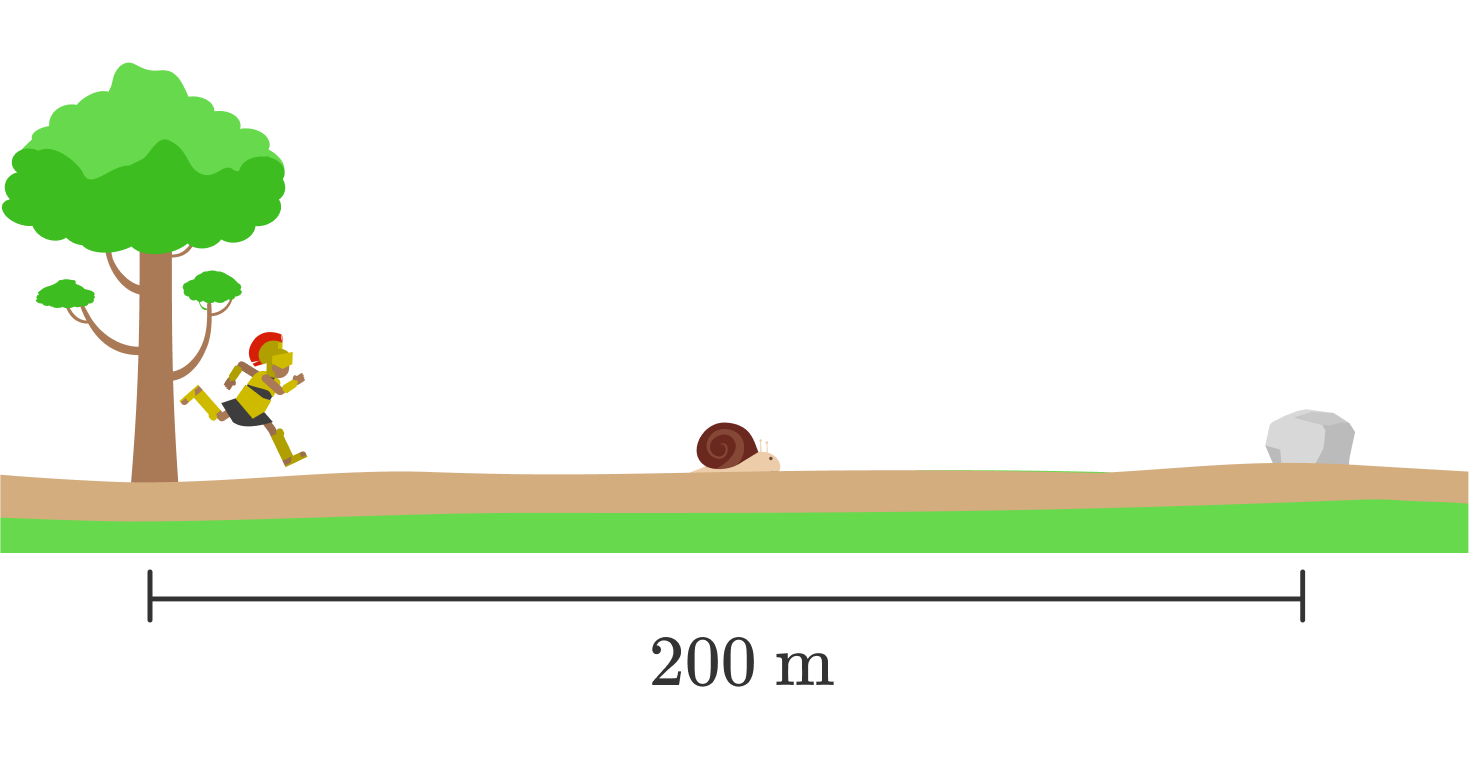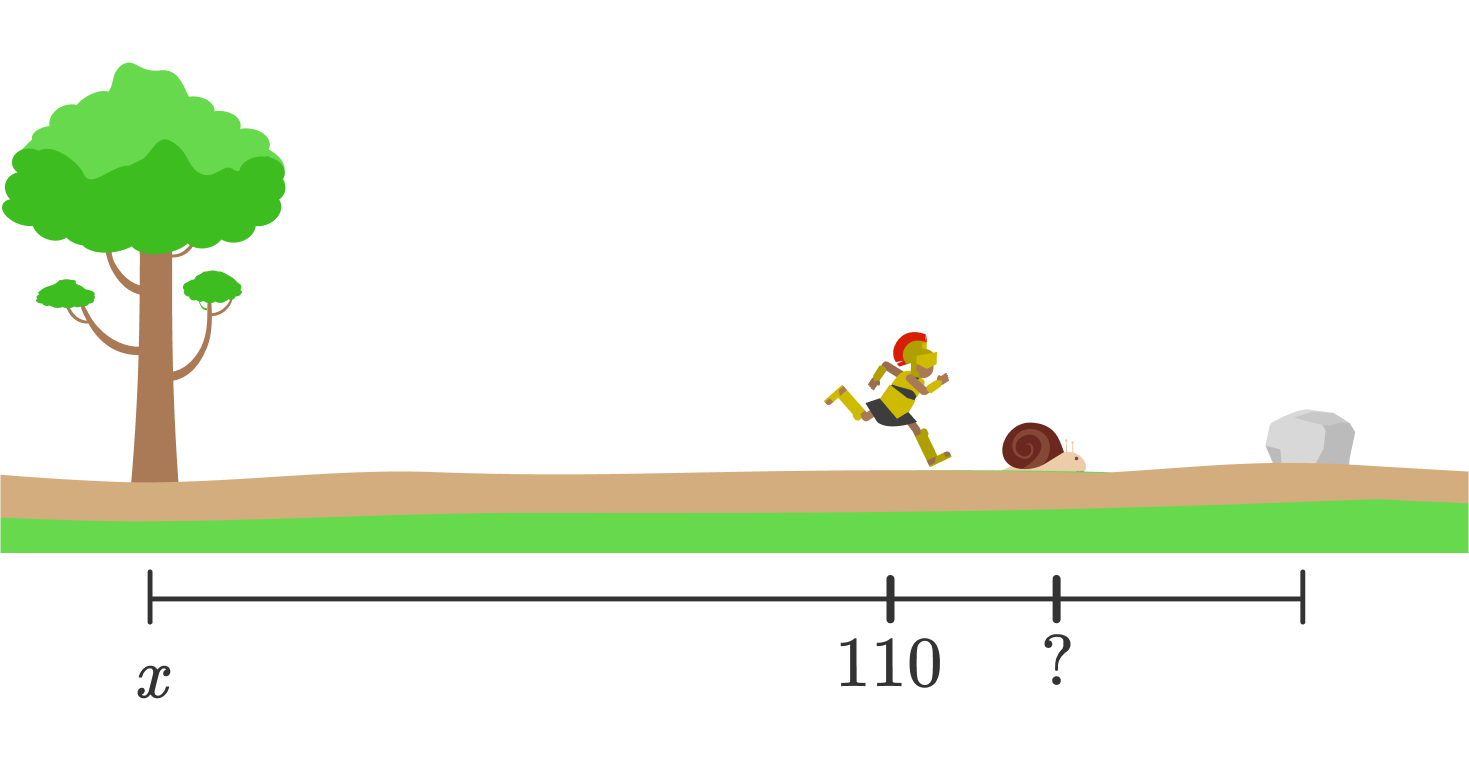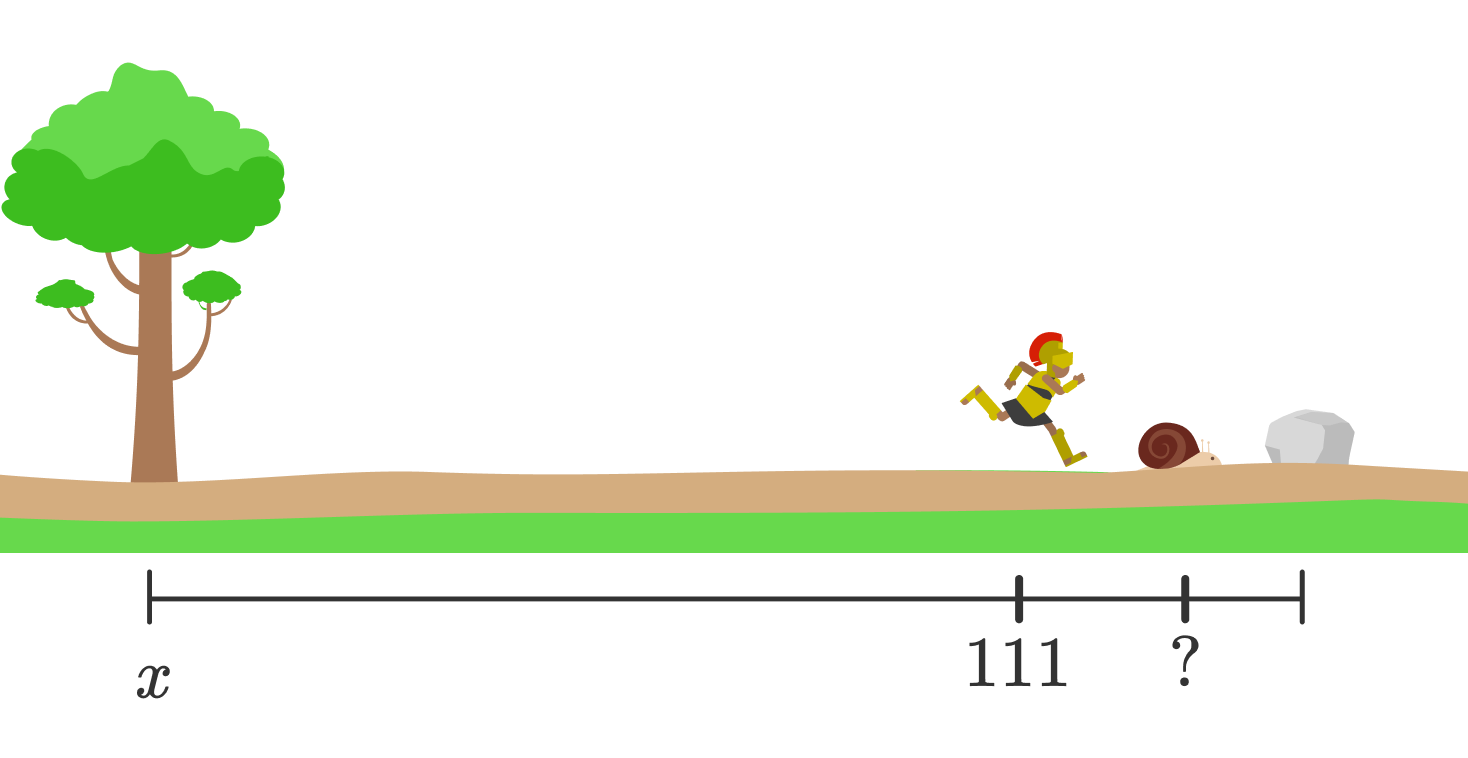### Integral Calculus

Calculus certainly has its practical aspects. It can help us find the extreme values of a function, compute volumes, probabilities, and a host of other scientific quantities.

It comes as a bit of a pleasant surprise that calculus can also resolve ancient philosophical riddles like Zeno's paradox of motion.

This quiz will guide you to a basic understanding of the paradox as a way of introducing infinite series.

Only basic algebra is needed for this quiz.

Achilles is the “fleetest foot of all mortals” — meaning he's a pretty fast runner. One day, a snail named Zeno challenges him to a race. “Achilles,” says the snail, “I'll wager the Golden Fleece that I can beat you in a race if you but give me a head start.”

Achilles, knowing he would look pretty good in that Fleece, responds, “You’re on! How can I possibly lose?”Achilles waits as Zeno makes his way to one half of the total 200-meter distance to the finish line at the grey stone.Let’s assume Achilles runs at a constant speed of 10 meters per second. We’ll also assume that Zeno runs at a rate of 1 meter per second, which is super fast for a snail!

After 10 seconds, where are Achilles and Zeno?

Note: Marks are measured away from the starting point, so the 90-meter mark is 90 meters from the start, for instance.

10 seconds into the race, Achilles is at the 100-meter mark and Zeno is at 110 meters. At what meter mark will Zeno be when Achilles makes it to the 110-meter mark?At this point in the race, Achilles starts to see why Zeno the snail was so certain he could win with a head start. By the time Achilles reaches a mark where Zeno was before, he still has farther to go.When Achilles reaches the 111-meter mark, where is Zeno?

It looks like the time it takes Achilles to catch up with Zeno is $10+ 1 + 0.1 + 0.01 + \cdots$ seconds, where the dots represent an infinite number of terms.

This problem comes from the Greek philosopher by the name of Zeno and is meant as a thought experiment. The heart of Zeno's paradox is the impossibility of completing an infinite sum.

This seems perfectly reasonable. After all, no matter how fast you are, adding two numbers together takes some amount of time. So it seems like adding an infinite number of numbers should take an infinite amount of time!

It took the invention of calculus, in particular, infinite series, to put Zeno's Paradox firmly to rest. We'll need much more machinery before we can show how calculus does this, but for the time being let's go ahead and figure out exactly how much time it takes Achilles to catch up to the snail.

Earlier, we found $x_{A}(t) = \left( \SI[per-mode=symbol]{10}{\meter\per\second}\right) t, \ x_{Z}(t) = \left( \SI[per-mode=symbol]{1}{\meter\per\second}\right) t + \SI{100}{\metre}.$ Using these functions, where $t$ is in seconds, compute the time (in seconds) it takes for Achilles to catch up to Zeno.

The resolution of Zeno's paradox comes from showing that $10 + 1 + 0.1 + 0.01 + \dots = 11.\bar{1} = \frac{100}{9}.$ In particular, we have to make sense of what it even means to sum an infinite number of numbers.

Achieving this will set us on a road to connect such infinite sums to topics like quantum theory, differential equations, and one of the most mysterious and useful formulas of all mathematics: Euler's formula.

The key notion underlying infinite series is the limit. In short, even though we can't add an infinite number of numbers, we can add a finite number of terms and then take the limit as the size of the sum goes to $\infty.$